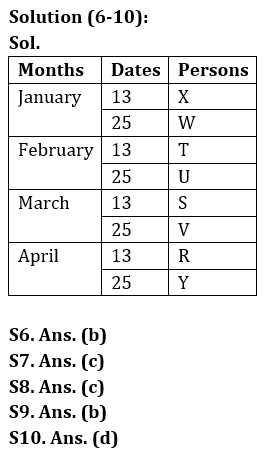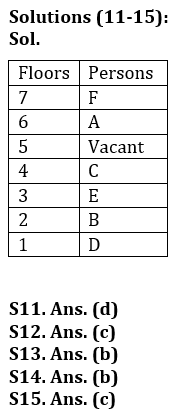Latest Banking jobs   »

# Reasoning Quiz For Bank of Baroda AO 2023-18th March

Directions (1-5): Study the following arrangement carefully and answer the questions given below:
3 1 2 5 4 7 2 8 4 5 7 1 9 6 5 2 4 1 4 5 8 2 4 3 9 7 6 9 4 2 3

Q1. Which of the following digit will be sixth to the left of tenth from the right end?
(a) 6
(b) 9
(c) 5
(d) 2
(e) None of these

Q2. How many 2’s is there in the given series which are immediately followed by a number less than 6?
(a) One
(b) None
(c) Three
(d) Two
(e) More than three

Q3. What will be the addition of fifth, seventh and tenth digit from the left end?
(a) 13
(b) 10
(c) 11
(d) 15
(e) None of these

Q4. If all the perfect square digits are removed from the given series (excluding 1), then which of the following will be fifth to the right of sixth from the left end?
(a) 5
(b) 2
(c) 8
(d) 1
(e) None of these

Q5. How many odd numbers are there in the given series which are immediately followed by a perfect square digit?
(a) One
(b) None
(c) Three
(d) Five
(e) Seven

Direction (6-10): Study the following information carefully and answer the questions given below:
Eight persons i.e., R, S, T, U, V, W, X and Y are working in an office. Each person takes one day leave in different days either on 13th or 25th of the four different months i.e., January, February, March and April but not necessarily in same order.
U takes leave before V but doesn’t take leave in the month of 31 days. There are three persons take leave between U and Y. R and S takes leave on prime numbered date. There are as many persons take leave before R as after W. Neither T nor X takes leave on 25th of the month. T takes leave before S and after X.

Q6. Who among the following takes leave on 13th April?
(a) X
(b) R
(c) T
(d) S
(e) None of these

Q7. How many persons take leave between Y and W?
(a) Three
(b) Two
(c) Five
(d) Four
(e) None of these

Q8. When V takes leave?
(a) 25th February
(b) 25th January
(c) 25th March
(d) 25th April
(e) None of these

Q9. Which among the following is true regarding X?
(a) No one takes leave after X
(b) Three persons take leave between X and S
(c) X takes leave on 25th January
(d) W takes leave before X
(e) None is true

Q10. Four of the following five are alike in certain way based from a group, find the one which does not belong to that group?
(a) S – 13th April
(b) V – 25th February
(c) U – 25th March
(d) W – 25th January
(e) U – 13th January

Direction (11-15) Read the information carefully and answer the questions given below:
Six people A, B, C, D, E, and F live on seven different floors of a building (such as ground floor numbered as 1 and top is numbered as 7 and 1 floor is vacant). E lives on an odd numbered floor. D lives on an odd numbered floor below E’s floor. F lives just above A, who doesn’t live on an odd numbered floor. F doesn’t live on 5th floor. C lives on even numbered floor above E’s floor. Numbers of floors between B and C is equal to numbers of floors between E and D.

Q11. Which of the following floor is vacant floor?
(a) second
(b) third
(c) fourth
(d) fifth
(e) None of these

Q12. Who among the following lives on third floor?
(a) C
(b) D
(c) E
(d) F
(e) None of these

Q13. B lives on which of the following floor?
(a) First
(b) Second
(c) Fourth
(d) Third
(e) None of these

Q14. How many persons are live between B and A?
(a) One
(b) Two
(c) Four
(d) Three
(e) None

Q15. Which of the following statement is not true about C?
(a) F lives one of the floors above C’s floor
(b) C lives on an even numbered floor
(c) C lives immediate above vacant floor
(d) Only One floor between C and A
(e) Both (b) and (d)

Solutions

Solution (1-5):
S1. Ans(d)
Sol. The 16th element from the right end = ‘2’.

S2. Ans(e)
Sol. There are four 2’s which are followed by number less than 6 i.e., ‘2 5, 2 4, 2 4 and 2 3’.

S3. Ans(c)
Sol. The fifth, seventh and tenth digit from the left end are ‘4, 2 and 5′.
Hence, the sum is = (4+2+5) = 11.

S4. Ans(b)
Sol. After removing all the perfect square digits ‘3 2 5 7 2 8 5 7 6 5 2 5 8 2 3 7 6 2 3’
Then (6+5) = 11th digit from the left is ‘2’.

S5. Ans(e)
Sol. There are seven odd digits which are followed by a perfect square number- ‘3 1, 5 4, 7 1, 1 9, 1 4, 3 9, 9 4’’## FAQs

### What is the selection process of Bank of Baroda AO 2023?

The selection process of Bank of Baroda AO consists of Online exam and Interview

#### Congratulations!Union Budget 2023-24: Free PDF